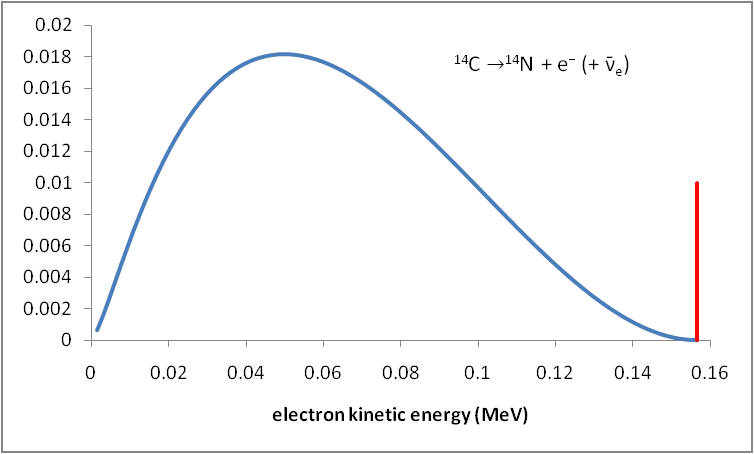# Why is beta decay continuous?

May 23, 2014

β decay is not continuous, but the kinetic energy spectrum of the emitted electrons is continuous.

β⁻ decay is a type of radioactive decay in which an electron is emitted from an atomic nucleus along with an electron antineutrino.Using symbols, we would write the β decay of carbon-14 as:Since the electrons are emitted as a stream of discrete particles, β decay is not continuous.

If you plot the fraction of electrons having a given kinetic energy against that energy, you get a graph like the one shown below.Emitted beta particles have a continuous kinetic energy spectrum. The energies range from 0 to the maximum available energy Q.

If only electrons carried away the energy, the graph would look like the red line at the right of the graph.

Instead, we get the continuous energy spectrum shown in blue.

The continuous energy spectrum occurs because Q is shared between the electron and the antineutrino.

A typical Q is around 1 MeV, but it can range from a few keV to a few tens of MeV. Since the rest mass energy of the electron is 511 keV, the most energetic β particles have speeds close to the speed of light.